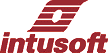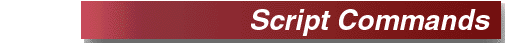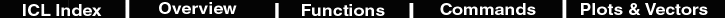ac Small-Signal Frequency Analysis Format: ac [dec] [oct] [lin] np fstart fstop Special Requirement: The AC analysis requires at least one voltage or current source in the circuit to have the AC magval (magnitude value) stimulus. For example: V1 1 0 AC 1 Examples: ac dec 10 1 10K ac oct 10 1k 100Meg ac lin 100 1 100HZ

 alias - generate an alias name for a vector Format: alias name vector or vector expression Example: alias vout v(8) alias rcurrent @r:1:test[i] alias output exp(v(8)*v(9))/((470/@d1[id])-1) An alias is used to give node voltages, instance current and power meaningful names. Schematic test points will create the correct alias syntax, equating the test point name with the correct quantity. Unalias is used to remove an alias. If the secod argument is an alias, its underlying vector is retrieved. You can use this feature to save vecor names for plotf.alter - change a value Format: alter @model[parameter] = value or expression alter @ref-des[parameter] = value or expression Example: alter @qn2222[bf] = 225 alter @r1[resistance] = @r1[resistance] + 10 alter @1:nmos[vto] = 2 Alter is used to change device/model parameters without leaving the simulator. Model is the name of the model whose parameter is to be changed. Instance parameters are shown in the part properties dialog for each part in your schematic. The key symbol defines model parameters.append - appends one vector to another Format: append tovec fromvec Example: append bigvec vec Takes one vector and appends it to another one.askvalues - prompts the user to enter a list of values Format: askvalues [ ] ... Example: skvalues val1 "First value" val2 "Second value" Displays a dialog entitled "Please provide the following values:". The body of the dialog contains a scrolling list containing the provided pairs of description strings and names, followed by edit windows in which the desired values can be entered. Entered values may be real or complex numbers, or valid expressions that can be evaluated to single or multi-element vectors. The resulting vectors, with the requested names, are added to the constants plot.assertvalid - verify the validity of specified vector names Format: assertvalid [] ... Example: assertvalid vin vout All listed vector names are evaluated to insure that they represent either existing vectors, or vectors which are available from the Add Waveforms dialog. If any vector is not found, an error message is posted, and the script is halted.autoscale - automatically rescales the traces of the active plot Format: autoscale [xy] Example: autoscale link xy All traces of the active plot are rescaled as specified. Allowable mode arguments are "link", "unlink", and "tile." If the optional second parameter "xy" is present, both the x and y axes are rescaled. If not, only the y axis is rescaled.Intuscope5 only.

 break - break from a loop Format: break Example: repeat 100 tran 1n 100n if mean(@d1[p]) > 50m break else alter @r1[resistance]=@r1[resistance]+10 end end If there is a while, dowhile, or repeat block enclosing this statement, control passes out of the block.compose - compose a vector Format: compose values .. Example: compose frequency values 10k+j(0) 500k+j(0) 5meg+j(0) 5.01meg+j(0) compose level values 90 54 54 60 Compose can be used to create real or complex vectors with user-specified values. These values can be arbitrary expressions. Note: Frequency must be defined as complex to get the proper plot type even though the imaginary part is almost always set to zero. The reason for this is that its values can have imaginary components and is how Spice3 extracts zeros in the pole zero analysis.copy - re-scale the current plot and copy the named vector Format: copy plot.vector Example: copy other.default copy tran2.v(5) Copy is used to bring a vector from another plot into the current plot and make it have a compatible scale. First, the current plot scale is combined with the other plots scale and all vectors in the current plot are interpolated to fit the new scale. The named vector is then interpolated the copied vector is named uniquely by appending it plot name, in the example tran2.v(5) would be v(5)#tran2 in the current plot. If the default keyword is used, nothing is copied but the current plot is re-scaled. See also newplot and for more information see plots and vectors.copytodoc - copies a trace from the current graph to another graph Format: copy plot.vector Example: copy other.default copy tran2.v(5) Copy is used to bring a vector from another plot into the current plot and make it have a compatible scale. First, the current plot scale is combined with the other plots scale and all vectors in the current plot are interpolated to fit the new scale. The named vector is then interpolated the copied vector is named uniquely by appending it plot name, in the example tran2.v(5) would be v(5)#tran2 in the current plot. If the default keyword is used, nothing is copied but the current plot is re-scaled. See also newplot and for more information see plots and vectors.Intuscope5 only.

 dc - DC Sweep Analysis Format: dc src start stop del [src2 start2 stop2 del2] Examples: dc VIN 0.25 5.0 0.25 dc VDS 0 10 .5 VGS 0 5 1 dc VCE 0 10 .25 IB 0 10U 1U Summary: The dc command is a special subset of IsSpice4's DC analysis features. It is used to perform a series of DC operating points by sweeping voltage and/or current sources and performing a DC operating point at each step value of the source(s). At each step, voltages, currents, and a variety of device/model parameters can be recorded. Syntax: The dc command defines the source to be swept and the sweep limits. Src is the name of an independent voltage or current source that will be swept. Start, stop, and del are the starting, final, and incrementing values, respectively. The first example will cause the value of the voltage source VIN to be swept from 0.25 Volts to 5.0 Volts in increments of 0.25 Volts. A second source (src2) may optionally be specified with associated sweep parameters (second example). In this case, the first source, VDS, will be swept over its range for each value of the second source, VGS. The dc command overrides any DC voltage specified in the actual voltage/current source statement. The DC voltage on the V or I line will be used during the AC and Transient analyses. But when the DC sweep is run, the values specified in the dc command will prevail.

 delete - destroy a breakpoint Format: delete [all] or [number] Deletes the specified breakpoint. Each breakpoint is assigned a unique number. These numbers can be found by using the status command. Use the stop command to set breakpoints.

 destroy - destroys a plot Format: destroy [all] [plotname] Examples: destroy plotname destroy all destroy Destroys the named plots or the current plot if there is no argument. The all keyword is permitted.destroyvec - deletes a waveform Format: destroyvec [[plotname.]vecname] ... Examples: destroyvec destroyvec plot1.v(1) destroyvec w1 w2 d Results: The active trace in the active plot is deleted. The trace of v(1) in plot1 is deleted. The traces with tag numbers 1 and 2 are deleted. Deletes the specified waveform trace or traces from the current graph document. If no traces are specified, the current trace in the active plot is deleted. If no plot is specified, either explicitly with a plot-name prefix, or implicitly by using the w notation, the trace must be in the active plot. Traces deleted using this command may be restored manally, by selecting "Undo" from the File menu.IntuScope only.

 diff - compare vectors from different plots Format: diff plotname1 plotname2 [vector] Example: diff tran1 tran2 v(8) Compares all vectors in the specified plots, or the vectors given, and if significant differences are found the differences are reported. The diff_abstol, diff_reltol, and diff_vntol variables are used to quantize the significant difference. The plots should have identical scales ( x-axis). Scales can be made the same using the copy command or the linearize command.display - list active vectors Format: display [vector] Example: display Prints a summary of the currently defined vectors, or of the vector(s) specified. The vectors are sorted by name unless the variable nosort is set. The summary contains the name, the length. The type, and whether it is a real or complex vector. One vector is label scale. This vector is used as the x axis scale when needed. See the setplot command to gain access to vector in other plots.disto - Small-Signal Distortion Analysis Format: disto [dec] [oct] [lin] np fstart fstop + [f2overf1] Examples: disto dec 10 1kHz 100MHz disto oct 10 1kHz 100Meg 0.9 disto lin 1 1MEG 100MHz 0.9 Special Requirement: The distortion analysis requires at least one voltage or current source in the circuit to have either the DISTOF1 or DISTOF2 keywords, or both. If the DISTOF1 or DISTOF2 keywords are missing from the description of an independent source, then that source is assumed to have no input at the corresponding frequency. The default values of the magnitude and phase are 1.0 and 0.0 degrees, respectively. At least one source in the circuit must have the DISTOF# stimulus in order to give the analysis meaning. For example: V1 1 0 DISTOF1 1 DISTOF2 0.01. See the IsSpice4 help for more information.

 dowhile - control loop Format: dowhile condition    commands end Examples: view tran v(3) function rms(vec) sqrt(mean(vec*vec)) dowhile rms(v(3)) > 400m     tran 1n 100n     alter @q5[temp]=@q5[temp]+10     print @q5[temp] rms(v(3)) end Note: Condition can be enclosed in parenthesis. The dowhile is functionally identical to the while loop. The difference is that the commands inside the loop are processed prior to the test of condition. See also while, break, repeat.echo - place text in the output file Format: echo [-n | -u | -nu | -un] text string Example: echo This is the sensitivity information Places the text given on the left into the output file for the given simulation. Text is left justified and ends with a new line unless the -n option is used. The -u option makes the text upper case.end - end of a loop or condition Format: end Example: while n > 5     n = n-1 end Terminate a loop or conditional clause. See also while, dowhile, repeat , if elseerrorstop - send error to task Format: errorstop program ID Example: while n > 5     n = n-1 end Halts a remote script on errors and sends the error message to the designated process. This should be used in connection with the automation interface of a remote process that connects to IsSpice4 using an ICL script.

 fftinit – initialize the FFT sin/cos table and plot space Format: fftinit radix Example: fftinit 13 timetofreq(current) alias previous = current dbmag = db(current) plotf dbmag dbmag(fft(%s)) previous phase = phase(current) plotf phase phase(fft(%s)) previous data = previous Attaches a time and frequency plot to the active plot. The number of points in these plots is set to 2^radix. The plots names have a "_f" suffix for frequency and a "_t" suffix for time. The frequency plot contains complex data; to plot the complex data, you should make and plot real vectors as shown in the example. See also timetofreq and freqtotime. Only one FFT radix is available for each plot. You can attach a different FFT radix to any other plot. The radix must be in the range of 3 (8points) to 25 (33.5 million points). You will need about 100Megs of RAM available for radix=20 (1million points) to keep from paging to your hard drive.plotf can only be used in scope5. The fft is available in IsSpice builds greater than 1628.

 filter - smoothes a vector Format: filter vector numpoints Example: filter v(32) 100 Filters a vector by numpoints using a triangular shaped weighted average. The triangle collapses at the endpoints. The base of the triangle is numpoints wide.foreach - loop for each value given Format: foreach var val1 val2 ... Example: foreach r1 10 20 30      tran 1n 100n      print pk_pk(vout) end OR foreach (r1 10 20 30)      tran 1n 100n      print pk_pk(vout) end Perform the instruction within the loop for each value given.fourier - Fourier analysis Format: fourier fundamental vector1... vectorn Example: fourier 10Meg v(8) v(7) i(vm1) The Fourier analysis is similar in functionality to the .FOUR command. It performs a Fourier analysis for each of the given values using the first 10 multiples of the fundamental requested. The vectors may be any saved vector or expression. Output is interpolated onto a fixed-space grid with the number of points given by the variable fourgridsize (default=200). The degree of interpolation is set by the polydegree variable (default=1). If polydegree is set to 0 no interpolation is performed. The number of harmonics displayed is determined by the nfreqs variable (default=10). All of the variables can be altered with the set command. The output is tabulated in the output file. Errors: An error message similar to "Wavelength longer than time span" will be generated it the transient analysis does not collect more than 1/freq data points.

 freqtotime - performs an FFT from frequency to time Format: freqtotime vector Example: freqtotime data     plot data Performs a fourier transform from frequency to time. If the plot hasn't been initialized using fftinit, it will set a default radix of 12, or 4096 points. See also fftinit and timetofreq.function - define a function Format: function function_name(args) expression Example: function max(x,y) (x > y) * x + (x <= y) * y function min(a,b,c) min(min(a,b),c) function rms(vec) sqrt(mean(vec*vec)) function sdev(vec) sqrt(mean(vec*vec) - mean(vec)^2) Defines a reusable function. The function can be used in any expression, for example, let test = max(v(8),v(3)).functionundef - undefine a function Format: functionundef [func_name] Example: functionundef max Deletes a user-defined function.goto - perform a jump Format: goto word Example: goto finish When a goto command is encountered control is transferred to the label word referenced by the goto command. You may jump out of a loop; however, it is illegal to jump into a loop.homecursors - initial cursors to the scale endpoints Format: homecursors ['view'] Example: homecursors" or "homecursors view" Purpose: Cursors 0 and 1 are respectively set either to the first and last data point in the default scale, or to the left and right side of the currently displayed graph plot. Remarks: If the optional parameter "view" is included, the cursors are set to the left and right sides of the plot. If there is no parameter, the cursors are set to the first and last data points. Scope Icon: The "view" parameter can only be used in Scope5.if - then - else - making a decision Format: if condition    commands else    commands end Example: if a > b    print a else    print b end Note: Condition can be enclosed in parenthesis, for example:     if(X>1) The else term and condition is optional. The if must be balanced with an end.isdisplayed Format: isdisplayed . Example: if isdisplayed (nv) Returns 1 if the argument is the name of a vector that is currently displayed as a trace in Scope, otherwise 0. If the argument is a simple vector name, the currently active plot is searched. If the argument is a plot name, vector name combination, for example, ., the specified plot is searched.- This symbol indicates that the script command is also used in IntuScope 5.